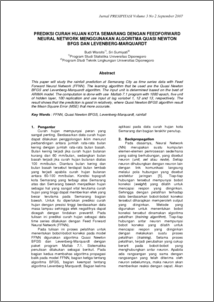# PREDIKSI CURAH HUJAN KOTA SEMARANG DENGAN FEEDFORWARD NEURAL NETWORK MENGGUNAKAN ALGORITMA QUASI NEWTON BFGS DAN LEVENBERG-MARQUARDT

Warsito, Budi and Ispriyanti, Dwi (2007) PREDIKSI CURAH HUJAN KOTA SEMARANG DENGAN FEEDFORWARD NEURAL NETWORK MENGGUNAKAN ALGORITMA QUASI NEWTON BFGS DAN LEVENBERG-MARQUARDT. Jurnal PRESIPITASI, 3 (2). pp. 1-7. ISSN 1907-187XPreview
PDF - Published Version
103Kb

Official URL: http://www.lingkungan.ft.undip.ac.id/

## Abstract

This paper will study the rainfall prediction at Semarang City as time series data with Feed Forward Neural Network (FFNN). The learning algorithm that be used are the Quasi Newton BFGS and Levenberg-Marquardt algorithm. The input unit is determined based on the best of ARIMA model. The computation is done with use Matlab 7.1 program with 1000 epoch, five unit of hidden layer, 100 replication and use input at lag variabel 1, 12 and 13, respectively. The result shows that the prediction is good in relatively, where Quasi Newton BFGS algorithm result the Mean Square Error (MSE) that more accurate. Key Words : FFNN, Quasi Newton BFGS, Levenberg-Marquardt, rainfall

Item Type: Article Q Science > Q Science (General) Faculty of Science and Mathematics > Department of Statistics 3506 INVALID USER 13 Jan 2010 14:54 13 Jan 2010 14:54

Repository Staff Only: item control page